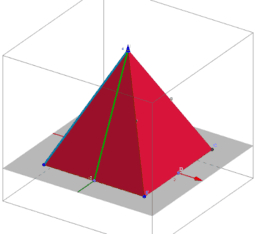# Area-Volumen-Algebra-inequalities-Trigonometric

Algebra - Trigonometric Functions Calculus Geometry :
•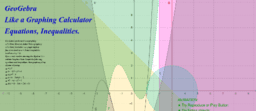### GeoGebra Like a Graphing Calculator Equations, Inequalities.

•### Graphing Trigonometric Functions: Sin

•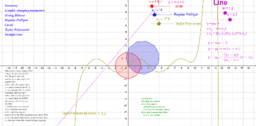### Geometry Graphic changing parameters-Using Sliders-1

•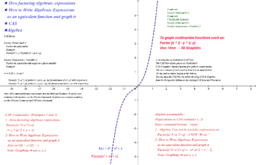### Factoring algebraic expressions: basic theory

•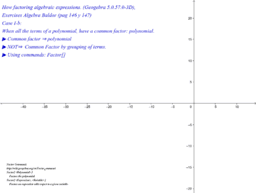### Factoring algebraic expressions: Common Factor, Polynomial

•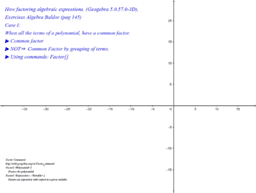### Factoring algebraic expressions: Common Factor, Baldor.

•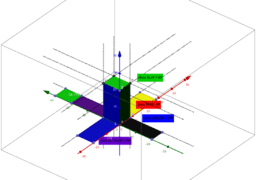### 1.4 Surface Area of Cubes and Rectangular Solids

•### 1.4 Surface Area and Volume of Cubes and Rectangular Solids

•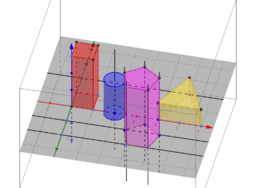### 1.4 Surface Area and Volume of Prisms and Cylinders

•